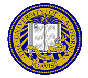# Homework 3

Points: 100
Due Date: Friday, November 12, 1999 at 11:59PM

1. (20 points) Chapter 1, exercise 4
2. (20 points) Chapter 1, exercise 9
3. (20 points) Chapter 2, exercise 4
4. (20 points) Find keys d and e for the RSA cryptosystem where p = 7 and q = 11.
5. (20 points) Chapter 20, exercise 4

## Extra Credit

1. (10 points) Chapter 2, exercise 17
2. (10 points) Chapter 20, exercise 5Send email to cs153@csif.cs.ucdavis.edu.

Department of Computer Science
University of California at Davis
Davis, CA 95616-8562